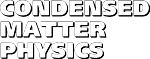Condensed Matter Physics, 2013, vol. 16, No. 2, 23003:1-10
DOI:10.5488/CMP.16.23003           arXiv:1304.4131

Title: The Wilson exact renormalization group equation and the anomalous dimension parameter
Author(s):
 C. Bervillier (Laboratoire de Mathematiques et Physique Theorique, UMR 7350 (CNRS), Federation Denis Poisson, Universite Francois Rabelais,CNRS, Parc de Grandmont, 37200 Tours, France)

The non-linear way the anomalous dimension parameter has been introduced in the historic first version of the exact renormalization group equation is compared to current practice. A simple expression for the exactly marginal redundant operator proceeds from this non-linearity, whereas in the linear case, first order differential equations must be solved to get it. The role of this operator in the construction of the flow equation is highlighted.

Key words: exact renormalization group equation, anomalous dimension
PACS: 05.10.Cc, 11.10.Gh, 64.60.ae

 Full text [pdf, ps] << List of papers